# 丁家山铅锌矿床三维可视化预测及其修正Three-Dimensional Visualization Prediction Model and Its Correction of Dingjiashan Pb-Zn Deposit

• 全文下载: PDF(3769KB)    PP.199-211   DOI: 10.12677/AG.2019.94023
• 下载量: 203  浏览量: 266

Aiming at the needs of deep prospecting in the Dingjiashan Pb-Zn deposit, a three-dimensional visualization prediction method was used to quantitatively predict the location of concealed ore bodies. By analyzing its ore-forming environment, metallogenic regularity and mineralization distribution law, a conceptual model of orebody location was established. The three-dimensional model of Dingjiashan geological bodies was established. The distance field analysis, slope analysis of geological interface and trend-undulation analysis of geological were used to extract ore-controlling factors such as distance field factors and unconformity surface trend-undulation factors. The nonlinear regression method was used to establish a three-dimensional prediction model of the ore body, and the Pb-Zn grades and metal amounts in the deep three-dimensional unit of the Dingjiashan Pb-Zn deposit were predicted. For the problem that the reliability of the prediction results decreases gradually with the increase of the prediction depth, this paper used the residual magnetic anomaly to modify the model, which help improve the reliability and accuracy of the three-dimensional prediction model of the concealed ore bodies.

1. 引言

2. 地质概况1-第四系；2-上侏罗统长林组；3、4、5-新元古界马面山岩群3-大岭组；4-龙北溪组上段；5-龙北溪组中段；6-花岗岩；7-石英斑岩；8-角度不整合界线；9-背斜；10-向斜；11-逆断层；12-压扭性断层；13-性质不明断层；14-地质界线；15-铅锌矿点；16-丁家山矿区范围

Figure 1. Simplified geological map of Dingjiashan Pb-Zn deposit

3. 矿体定位概念模型Table 1. Conceptual model for ore body location

4. 控矿地质因素定量提取

4.1. 不整合面距离场因素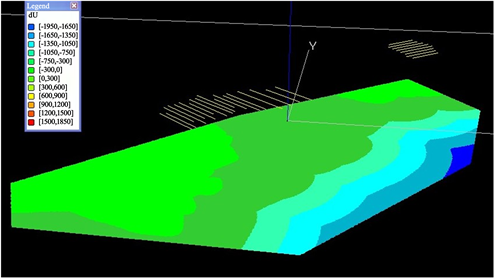Figure 2. Unconformity surface distance field factor (dU)

4.2. 不整合面趋势–起伏因素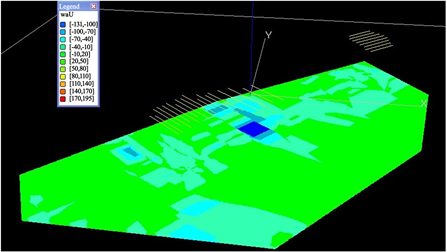Figure 3. First level undulation of unconformity surface (waU)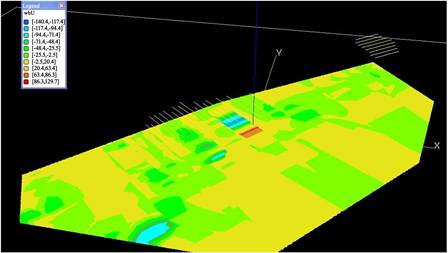Figure 4. Second level undulation of unconformity surface (wbU)

4.3. 不整合面坡度因素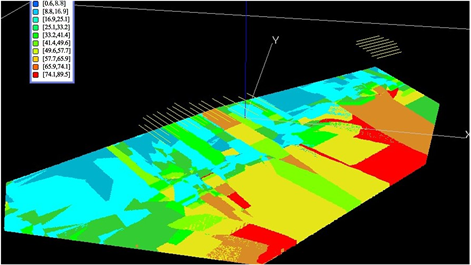Figure 5. Slope factor of unconformity surface (gU)

4.4. 不整合面夹角因素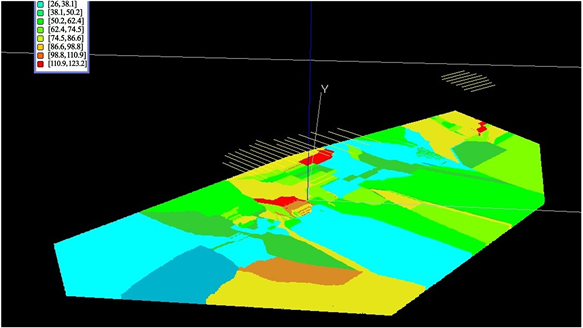Figure 6. Angle factor of unconformity surface (aU_S)

5. 三维预测模型建立

$MVk=Bk0+\underset{j=1}{\overset{p}{\sum }}BkjGVj+\epsilon k=1,2,3,\dots ,n$ (1)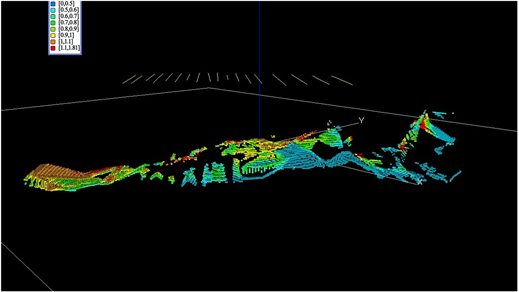Figure 7. Prediction of Pb grade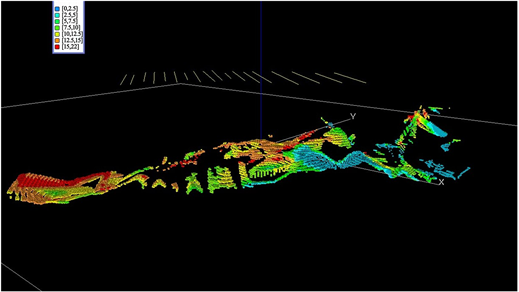Figure 8. Prediction of Pb metal quantity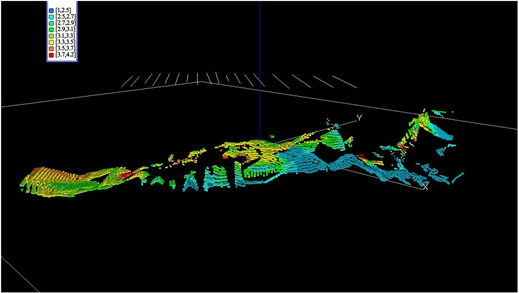Figure 9. Prediction of Zn grade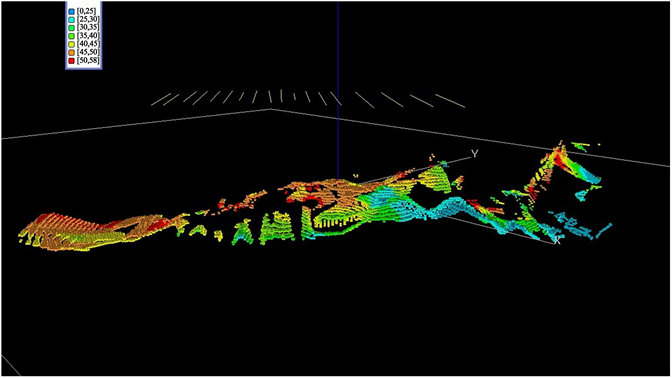Figure 10. Prediction of Zn metal quantity

6. 磁异常驱动的预测模型修正

6.1. 地质模型转换为物性模型

1) 根据磁化率求取感应磁化强度(Ji)，Ji= kT0。其中T0为当地磁场，k为磁化率，参考测定统计的岩(矿)石磁性资料报告获得。据梅仙矿区勘察高精度磁测项目报告，当地磁场大小约为46368.5 nT，其中银铅锌矿的物性参数为k = 4π × 4490 × 10−6 SI，矿化绿片岩的物性参数为k = 4π × 4490 × 10−6 SI。

2) 获取剩余磁化强度(Jr)，参考测定统计的岩(矿)石磁性资料报告获得。根据福建省尤溪县梅仙矿区勘察高精度磁测项目报告，项目研究中分为三种情况：

a) Pd ≥ 0.5%或Zn ≥ 1%，且(PbMet + ZnMet) > 0，则有Jr = 2.689 A/m。

b) 当条件不满足条件1时，且Pb > 0或Zn > 0，则有Jr = 0.144 A/m。

c) 当条件1和2都不满足时，则有Jr = 0 A/m。

3) 求总磁化强度(J)，J = Ji + Jr

4) 数据坐标整理，使其符合正演程序输入规则(表2)。Table 2. Geological object physical property parameters

6.2. 磁异常三维正演模拟

$\Delta T={H}_{ax}\mathrm{cos}{I}_{0}\mathrm{cos}{{A}^{\prime }}_{0}+{H}_{ay}\mathrm{cos}{I}_{0}\mathrm{sin}{{A}^{\prime }}_{0}+{Z}_{a}\mathrm{sin}{I}_{0}$ (2)

${H}_{ax}=\frac{{\mu }_{0}}{4\text{π}}\underset{\upsilon }{\int }M×\left\{\begin{array}{l}\frac{2{\left(x-\xi \right)}^{2}-{\left(y-\eta \right)}^{2}-{\left(z-\zeta \right)}^{2}}{{r}^{5}}\mathrm{cos}I\mathrm{sin}A+\frac{3\left(x-\xi \right)\left(y-\eta \right)}{{r}^{5}}\mathrm{cos}I\mathrm{cos}A\\ +\frac{3\left(x-\xi \right)\left(z-\zeta \right)}{{r}^{5}}\mathrm{sin}I\end{array}\right\}\text{d}\upsilon$ (3)

${H}_{ay}=\frac{{\mu }_{0}}{4\text{π}}\underset{\upsilon }{\int }M×\left\{\begin{array}{l}\frac{3\left(x-\xi \right)\left(y-\eta \right)}{{r}^{5}}\mathrm{cos}I\mathrm{sin}A+\frac{2{\left(y-\eta \right)}^{2}-{\left(x-\xi \right)}^{2}-{\left(z-\zeta \right)}^{2}}{{r}^{5}}\mathrm{cos}I\mathrm{cos}A\\ +\frac{3\left(y-\eta \right)\left(z-\zeta \right)}{{r}^{5}}\mathrm{sin}I\end{array}\right\}\text{d}\upsilon$ (4)

${Z}_{a}=\frac{{\mu }_{0}}{4\text{π}}\underset{\upsilon }{\int }M×\left\{\begin{array}{l}\frac{3\left(x-\xi \right)\left(z-\zeta \right)}{{r}^{5}}\mathrm{cos}I\mathrm{sin}A+\frac{3\left(z-\zeta \right)\left(y-\eta \right)}{{r}^{5}}\mathrm{cos}I\mathrm{cos}A\\ +\frac{2{\left(z-\zeta \right)}^{2}-{\left(x-\xi \right)}^{2}-{\left(y-\eta \right)}^{2}}{{r}^{5}}\mathrm{sin}I\end{array}\right\}\text{d}\upsilon$ (5)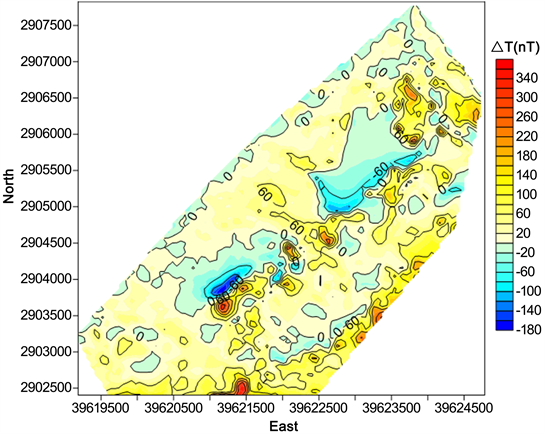Figure 11. Magnetic anomaly forward results of Dingjiashan model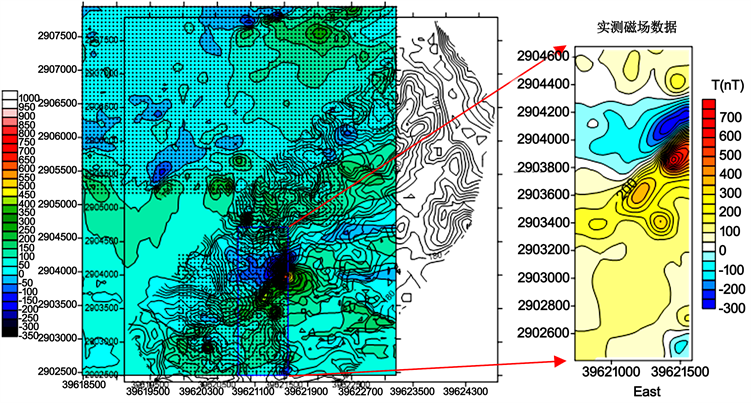Figure 12. Research area

6.3. 预测模型修正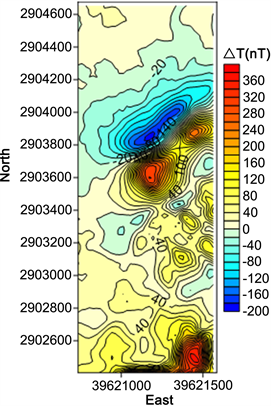(a) 模型正演磁异常(b) 正演结果与实测数据之差

Figure 13. Magnetic anomaly of research area: (a) Model magnetic anomaly forward results; (b) Residual magnetic anomaly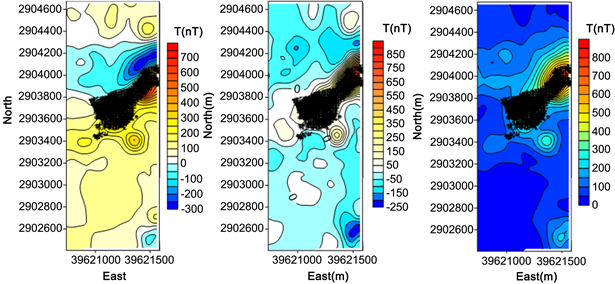Figure 14. Horizontal projection of ore body

1) 研究区域右下方应该不存在矿体，此区域实测的负异常是由研究区域右下方的矿体产生，不在建模范围内，因此不用考虑。

2) 坐标大致为(39621500，2903900)处的矿体的体积应扩大，并且其下方和右下方可能存在比已知矿体更大一些的隐伏矿体。

3) 坐标(39621350，2903390)处应该存在一个小规模矿体，其产生的正异常会保存，而负异常会被其上方的矿体产生的正异常所覆盖。

7. 结论

1) 本文按照“地质信息集成–成矿信息定量提取–立体定量预测”的流程，对丁家山铅锌矿床深部体元的品位及金属量进行预测，为深部找矿提供三维可视化定位定量预测。

2) 针对隐伏矿体三维预测随着预测深度的逐渐增加，预测准确率降低的问题，本文加入了基于磁异常驱动的迭代修正步骤，对隐伏矿体三维预测模型进行修正，使预测模型更接近真实地质结构。

  虞建平, 李桂秀, 刘小胡, 等. 丁家山铅锌矿床稀土元素地球化学特征研究[J]. 中国稀土学报, 2013, 31(3): 368-379.  陈小华. 福建省丁家山铅锌矿床地质特征及成因[J]. 福建地质, 2000(2): 57-65.  陈建平, 吕鹏, 吴文, 等. 基于三维可视化技术的隐伏矿体预测[J]. 地学前缘, 2007, 14(5): 54-62.  李秋金, 李金梅, 王芳. 闽中梅仙铅锌矿田赋矿变质岩系对比及深部找矿前景[J]. 地质学刊, 2016, 40(2): 234-242.  毛先成. 三维数字矿床与隐伏矿体立体定量预测研究[D]: [博士学位论文]. 长沙: 中南大学, 2006.  毛先成, 邹艳红, 陈进, 等. 危机矿山深部边部隐伏矿体的三维可视化预测——以安徽铜陵凤凰山矿田为例[J]. 地质通报, 2010, 29(2): 401-413.  Arndt, N.T., Lesher, C.M. and Czamanske, G.K. (2005) Mantle-Derived Magmas and Magmatic Ni-Cu-(PGE) Deposits. Economic Geology, 5-24.  林玉婷, 毛先成, 刘晓霞, 等. 新疆阿希金矿控矿因素三维定量分析与提取[J]. 地质学刊, 2016, 40(3): 477-485.  Chai, G. and Naldrett, A.J. (1992) Characteristics of Ni-Cu-PGE Mineralization and Genesis of the Jinchuan Deposit, Northwest China. Economic Geology, 87, 1475-1495. https://doi.org/10.2113/gsecongeo.87.6.1475  Chen, L.M., Song, X.Y., Keays, R.R., et al. (2013) Segregation and Fractionation of Magmatic Ni-Cu-PGE Sulfides in the Western Jinchuan Intrusion, Northwestern China: Insights from Platinum Group Element Geochemistry. Economic Geology, 108, 1793-1811. https://doi.org/10.2113/econgeo.108.8.1793  肖克炎, 朱裕生, 张晓华, 等. 矿产资源评价中的成矿信息提取与综合技术[J]. 矿床地质, 1999(4): 379-384.  Li, C., Ripley, E.M. and Naldrett, A.J. (2009) A New Genetic Model for the Giant Ni-Cu-PGE Sulfide Deposits Associated with the Siberian Flood Basalts. Economic Geology, 104, 291-301. https://doi.org/10.2113/gsecongeo.104.2.291  严加永, 滕吉文, 吕庆田. 深部金属矿产资源地球物理勘查与应用[J]. 地球物理学进展, 2008, 23(3): 871-891.  Krige, D.G. and Magri, E.J. (1982) Studies of the Effects of Outliers and Data Transformation on Variogram Estimates for a Base Metal and a Gold Ore Body. Mathematical Geology, 14, 557-564. https://doi.org/10.1007/BF01033879  黄继先, 毛先成, 陈进, 等. 基于GWR的丁家山铅锌矿控矿因素影响研究[J]. 地质学刊, 2017, 41(3): 401-408.  Houlding, S. (1994) 3D Geoscience Modeling: Computer Techniques for Geological Characterization. Springer Science & Business Media, Berlin. https://doi.org/10.1007/978-3-642-79012-6  李朝阳, 刘玉平, 管太阳, 等. 不整合面中的成矿机制与找矿研究[J]. 地学前缘, 2004(2): 353-360.  车申, 谢其锋, 龚孔成, 等. 福建省丁家山铅锌矿床地球物理特征及深部找矿前景[J]. 中国矿业, 2015, 24(11): 89-94.  廖崇高, 杨武年, 刘登忠, 等. 基于GIS空间分析进行多源信息成矿预测[J]. 物探化探计算技术, 2002, 24(2): 146-150.  邹艳红, 周胜, 陈进, 等. 基于3维可视化环境的地质体空间形态分析[J]. 地理信息世界, 2008, 6(5): 33-38.  Eppinger, R.G., Fey, D.L., Giles, S.A., et al. (2013) Summary of Exploration Geochemical and Mineralogical Studies at the Giant Pebble Porphyry Cu-Au-Mo Deposit, Alaska: Implications for Exploration under Cover. Economic Geology, 108, 495-527. https://doi.org/10.2113/econgeo.108.3.495  Evans-Lamswood, D.M., Butt, D.P., Jackson, R.S., et al. (2000) Physical Controls Associated with the Distribution of Sulfides in the Voisey’s Bay Ni-Cu-Co Deposit, Labrador. Economic Geology, 95, 749-769. https://doi.org/10.2113/95.4.749  黄继先, 毛先成, 邓浩. 一种面向三维地质剖面的形体表面重构算法[J]. 地理与地理信息科学, 2017, 33(1): 19-22, 34.  毛先成, 张苗苗, 邓浩, 等. 矿区深部隐伏矿体三维可视化预测方法[J]. 地质学刊, 2016, 40(3): 363-371.  强建科, 李俊营, 鲁凯, 等. 井巷环境下微重力异常研究[J]. 地球物理学进展, 2018, 33(5): 1-6.  吴乐园. 重磁位场频率域高精度正演方法: Gauss-FFT法[D]: [博士学位论文]. 杭州: 浙江大学, 2014.  强建科, 满开峰, 鲁凯, 等. 基于虚拟背景场的相对感应电动势研究[J]. 地球物理学进展, 2016, 31(4): 1655-1659.  强建科, 周俊杰, 满开峰. 时间域航空电磁法2.5维有限元模拟[J]. 物探与化探, 2015, 39(5): 1059-1062.  韩雪, 强建科, 鲁凯, 等. 基于二维数据的加权非线性共轭梯度三维反演[J]. 工程地球物理学报, 2016, 13(5): 561-569.  Journel, A.G. and Huijbregts, C.J. (1978) Mining Geostatistics. Academic Press, Cambridge.  祁光, 吕庆田, 严加永, 等. 基于先验信息约束的三维地质建模:以庐枞矿集区为例[J]. 地质学报, 2014, 88(4): 466-477.  潘懋, 方裕, 屈红刚. 三维地质建模若干基本问题探讨[J]. 地理与地理信息科学, 2007(3): 1-5.  祁光, 吕庆田, 严加永, 等. 先验地质信息约束下的三维重磁反演建模研究——以安徽泥河铁矿为例[J]. 地球物理学报, 2012, 55(12): 4194-4206.  Chai, G. and Naldrett, A.J. (1992) The Jinchuan Ultramafic Intrusion: Cumulate of a High-Mg Basaltic Magma. Journal of Petrology, 33, 277-303. https://doi.org/10.1093/petrology/33.2.277